## Python Tutorial

Python HOME Python Intro Python Get Started Python Syntax Python Comments Python Variables Python Data Types Python Numbers Python Casting Python Strings Python Booleans Python Operators Python Lists Python Tuples Python Sets Python Dictionaries Python If...Else Python While Loops Python For Loops Python Functions Python Lambda Python Arrays Python Classes/Objects Python Inheritance Python Iterators Python Scope Python Modules Python Dates Python Math Python JSON Python RegEx Python PIP Python Try...Except Python User Input Python String Formatting

## File Handling

Python File Handling Python Read Files Python Write/Create Files Python Delete Files

## Python Modules

NumPy Tutorial Pandas Tutorial SciPy Tutorial Django Tutorial

## Python Matplotlib

Matplotlib Intro Matplotlib Get Started Matplotlib Pyplot Matplotlib Plotting Matplotlib Markers Matplotlib Line Matplotlib Labels Matplotlib Grid Matplotlib Subplot Matplotlib Scatter Matplotlib Bars Matplotlib Histograms Matplotlib Pie Charts

## Machine Learning

Getting Started Mean Median Mode Standard Deviation Percentile Data Distribution Normal Data Distribution Scatter Plot Linear Regression Polynomial Regression Multiple Regression Scale Train/Test Decision Tree Confusion Matrix Hierarchical Clustering Logistic Regression Grid Search Categorical Data K-means Bootstrap Aggregation Cross Validation AUC - ROC Curve K-nearest neighbors

## Python MySQL

MySQL Get Started MySQL Create Database MySQL Create Table MySQL Insert MySQL Select MySQL Where MySQL Order By MySQL Delete MySQL Drop Table MySQL Update MySQL Limit MySQL Join

## Python MongoDB

MongoDB Get Started MongoDB Create Database MongoDB Create Collection MongoDB Insert MongoDB Find MongoDB Query MongoDB Sort MongoDB Delete MongoDB Drop Collection MongoDB Update MongoDB Limit

## Python Reference

Python Overview Python Built-in Functions Python String Methods Python List Methods Python Dictionary Methods Python Tuple Methods Python Set Methods Python File Methods Python Keywords Python Exceptions Python Glossary

## Module Reference

Random Module Requests Module Statistics Module Math Module cMath Module

## Python How To

Remove List Duplicates Reverse a String Add Two Numbers

## Python Examples

Python Examples Python Compiler Python Exercises Python Quiz Python Certificate

# Matplotlib Bars

## Creating Bars

With Pyplot, you can use the `bar()` function to draw bar graphs:

### Example

Draw 4 bars:

import matplotlib.pyplot as plt
import numpy as np

x = np.array(["A", "B", "C", "D"])
y = np.array([3, 8, 1, 10])

plt.bar(x,y)
plt.show()

### Result: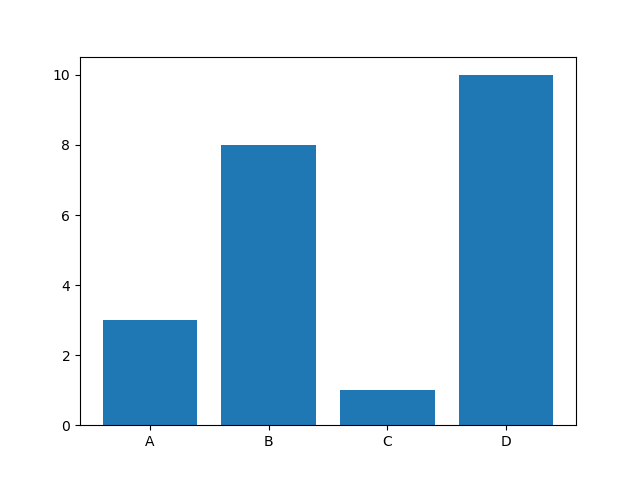Try it Yourself »

The `bar()` function takes arguments that describes the layout of the bars.

The categories and their values represented by the first and second argument as arrays.

### Example

x = ["APPLES", "BANANAS"]
y = [400, 350]
plt.bar(x, y)

## Horizontal Bars

If you want the bars to be displayed horizontally instead of vertically, use the `barh()` function:

### Example

Draw 4 horizontal bars:

import matplotlib.pyplot as plt
import numpy as np

x = np.array(["A", "B", "C", "D"])
y = np.array([3, 8, 1, 10])

plt.barh(x, y)
plt.show()

### Result:Try it Yourself »

## Bar Color

The `bar()` and `barh()` takes the keyword argument `color` to set the color of the bars:

### Example

Draw 4 red bars:

import matplotlib.pyplot as plt
import numpy as np

x = np.array(["A", "B", "C", "D"])
y = np.array([3, 8, 1, 10])

plt.bar(x, y, color = "red")
plt.show()

### Result: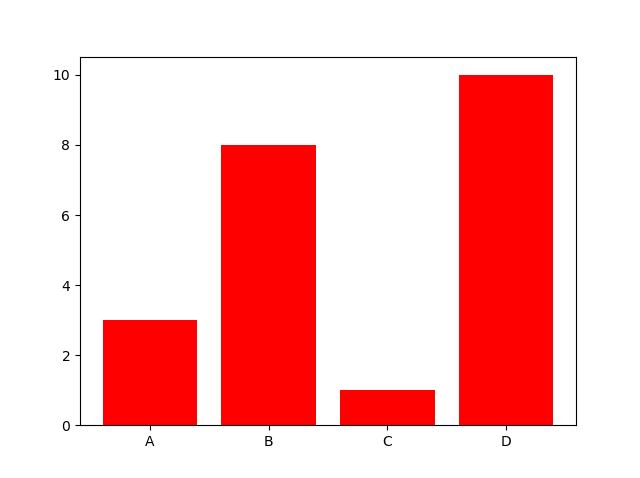Try it Yourself »

### Color Names

You can use any of the 140 supported color names.

### Example

Draw 4 "hot pink" bars:

import matplotlib.pyplot as plt
import numpy as np

x = np.array(["A", "B", "C", "D"])
y = np.array([3, 8, 1, 10])

plt.bar(x, y, color = "hotpink")
plt.show()

### Result: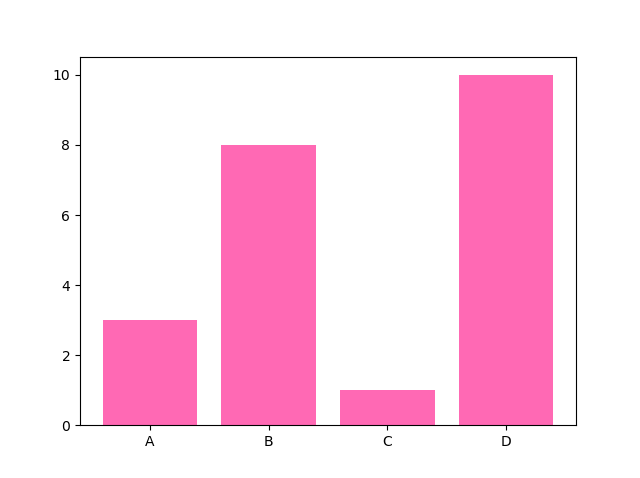Try it Yourself »

### Color Hex

Or you can use Hexadecimal color values:

### Example

Draw 4 bars with a beautiful green color:

import matplotlib.pyplot as plt
import numpy as np

x = np.array(["A", "B", "C", "D"])
y = np.array([3, 8, 1, 10])

plt.bar(x, y, color = "#4CAF50")
plt.show()

### Result: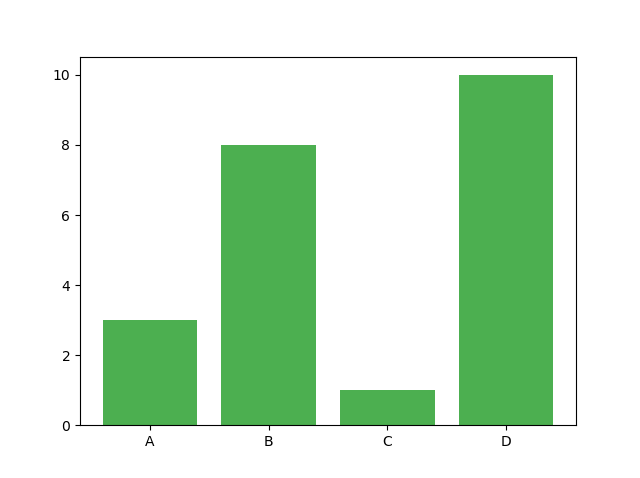Try it Yourself »

## Bar Width

The `bar()` takes the keyword argument `width` to set the width of the bars:

### Example

Draw 4 very thin bars:

import matplotlib.pyplot as plt
import numpy as np

x = np.array(["A", "B", "C", "D"])
y = np.array([3, 8, 1, 10])

plt.bar(x, y, width = 0.1)
plt.show()

### Result: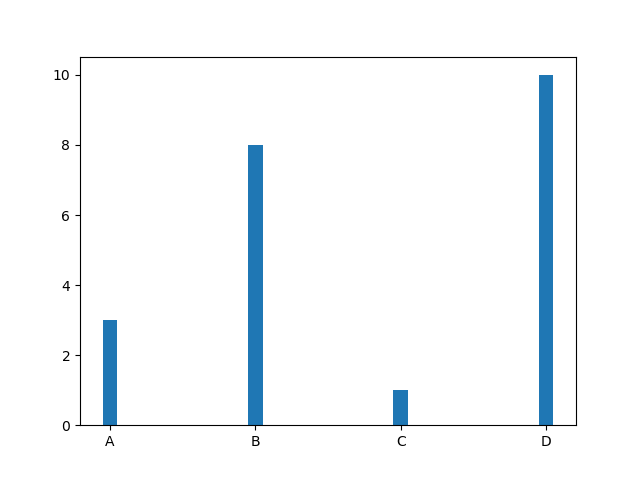Try it Yourself »

The default width value is 0.8

Note: For horizontal bars, use `height` instead of `width`.

## Bar Height

The `barh()` takes the keyword argument `height` to set the height of the bars:

### Example

Draw 4 very thin bars:

import matplotlib.pyplot as plt
import numpy as np

x = np.array(["A", "B", "C", "D"])
y = np.array([3, 8, 1, 10])

plt.barh(x, y, height = 0.1)
plt.show()

### Result: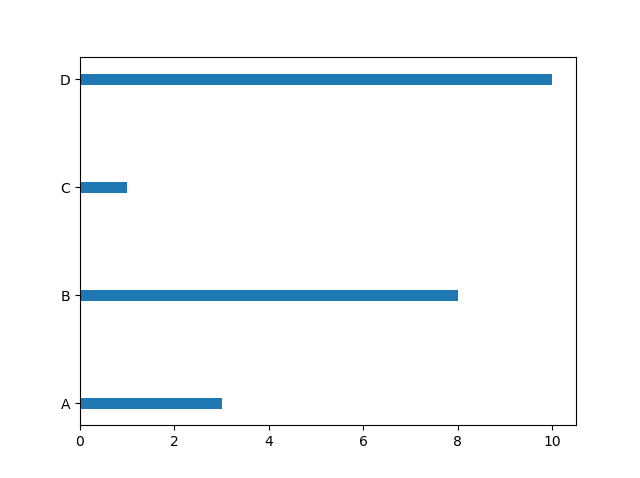Try it Yourself »

The default height value is 0.8